# Math in Focus Grade 2 Chapter 5 Practice 3 Answer Key How to Divide

Go through the Math in Focus Grade 2 Workbook Answer Key Chapter 5 Practice 3 How to Divide to finish your assignments.

## Math in Focus Grade 2 Chapter 5 Practice 3 Answer Key How to Divide

Example

Divide 10 snails into 2 equal groups.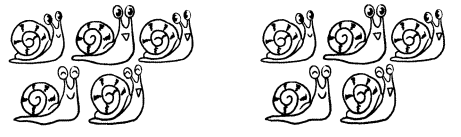10 ÷ 2 = 5
There are 5 snails ¡n each group.

Question 1.
Divide 15 books into 3 equal stacks.15 ÷ ______ = ______
There are ________ books in each stack.
Given that number of books are 15
The 15 books are divided into 3 equal stacks.
Number of books in each stack is 15 ÷ 3 = 5
There are 5 books in each stack.

Question 2.
Divide 15 eggs equally info 5 nests.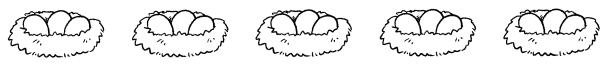15 ÷ ______ = ______
There are ___ eggs in each nest.
Given that there are 15 eggs.
15 eggs are divided into 5 nests.
Number of eggs in each nest is 15 ÷ 5 = 3
There are 3 eggs in each nest.

Find the number of groups.
Fill in the blanks.

Example

Divide 15 pancakes so there are 3 pancakes in each group.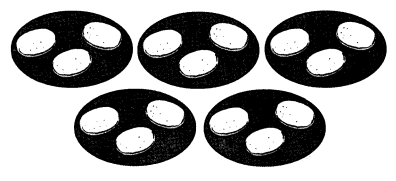Subtract groups of 3 until there is nothing left.
15 ÷ 33333 = 0
How many times do you subtract groups of 3? 5
15 ÷ 3 = 5
There are 5 groups.

Question 3.
Divide 12 beads into groups of 4.
12 – ______ – ___ – ___ = 0
12 ÷ ___ = ____
There are ___ groups of 4 beads.
Given that number of beads are 12.
They are divided into 4 groups.
12 – 4 – 4 – 4 = 0
12 ÷ 4 = 3
There are 3 groups of 4 beads.

Question 4.
Divide 14 frozen yogurt cones into groups of 7.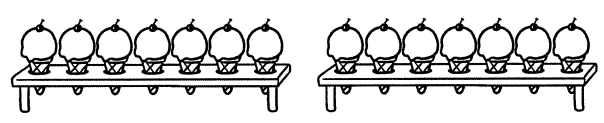14 – ___ – ___ = 0
14 ÷ ___ = ____
There are ___ groups of 7 frozen yogurt cones.
Given that there are 14 frozen yogurt cones.
These 14 frozen yogurt cones are 7 of each group.
Number of groups are
14 – 7 – 7 = 0
14 ÷ 2 = 7
There are 2 groups of 7 frozen yoghurt cones.

Question 5.
Put 20 oranges onto plates with 5 on each plate.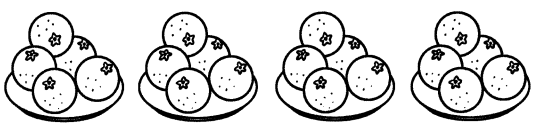20 – _______ – _______ — _______ — _______ =0
20 ÷ ______ = ______
There are ___ plates of 5 oranges.

Given that total number of oranges are 20
The 20 oranges are put in a 5 plates.
Number of oranges on each plate are
20 – 5 – 5 – 5 – 5 = 0
20 ÷ 5 = 4
There are 4 plates of 5 oranges.

Fill in the blanks.

Question 6.
Put 16 glasses onto trays with 4 on each tray.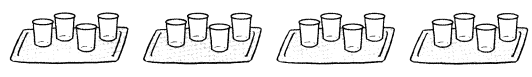16 – _______ — _______ — _______ — _______ = 0
16 ÷ ______ = ______
There are ___ trays of 4 glasses.
Given that there are 16 glasses.
Each tray has 4 glasses.
Number of trays are
16 – 4 – 4 – 4 – 4 = 0
16 ÷ 4 = 4
There are 4 trays of 4 glasses.

Question 7.
Put 8 cookies onto plates with 2 on each tray.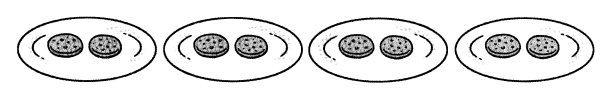8 – ___ – ___ – ____ – ___ = 0
8 ÷ ___ = ____
There are ___ plates of 2 cookies.